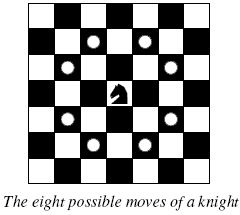# Competitive Programming: POJ 2488 – A Knight’s Journey

### Description

#### BackgroundThe knight is getting bored of seeing the same black and white squares again and again and has decided to make a journey around the world. Whenever a knight moves, it is two squares in one direction and one square perpendicular to this. The world of a knight is the chessboard he is living on. Our knight lives on a chessboard that has a smaller area than a regular 8 * 8 board, but it is still rectangular. Can you help this adventurous knight to make travel plans?

#### Problem

Find a path such that the knight visits every square once. The knight can start and end on any square of the board.

### Input

The input begins with a positive integer n in the first line. The following lines contain n test cases. Each test case consists of a single line with two positive integers p and q, such that 1 <= p * q <= 26. This represents a p * q chessboard, where p describes how many different square numbers 1, . . . , p exist, q describes how many different square letters exist. These are the first q letters of the Latin alphabet: A, . . .

### Output

The output for every scenario begins with a line containing “Scenario #i:”, where i is the number of the scenario starting at 1. Then print a single line containing the lexicographically first path that visits all squares of the chessboard with knight moves followed by an empty line. The path should be given on a single line by concatenating the names of the visited squares. Each square name consists of a capital letter followed by a number.
If no such path exist, you should output impossible on a single line.

```3
1 1
2 3
4 3
```

### Sample Output

```Scenario #1:
A1

Scenario #2:
impossible

Scenario #3:
A1B3C1A2B4C2A3B1C3A4B2C4
```

Solution below . . .

```class KnightTour {
static int H, W;

/*
* Check if x,y are within the bounds of an H*W chessboard
*/
static boolean isSafe(int x, int y, int sol[][]) {
return (x >= 0 && x < H && y >= 0 && y < W && sol[x][y] == -1);
}

/*
* A utility function to print solution in the desired format
*/
static void printSolution(int sol[][]) {
String[] solution = new String[H * W];

/*
* The solution matrix (sol[][]) contains the solution as a
* sequence of numbers from 0 to H * W - 1, showing the order
* in which each square is visited. We need to convert that
* to the output format specified by the problem description.
*/
for (int x = 0; x < H; x++) {
for (int y = 0; y < W; y++) {
char row = (char) ('A' + y);
int col = x + 1;
solution[sol[x][y]] = "" + row + col;
}
}

StringBuilder sb = new StringBuilder();

for (String s : solution) {
sb.append(s);
}

System.out.println(sb.toString());
}

/*
* This function solves the Knight Tour problem using backtracking. Backtracking
* is not the most efficient solution but it does let us control the order in
* which squares are visited. This is important because there can be multiple
* solutions and the problem asks for the lexicographically first path.
*/
static boolean solveKT(int test) {
int sol[][] = new int[H][W];

/* Initialization of solution matrix */
for (int x = 0; x < H; x++)
for (int y = 0; y < W; y++)
sol[x][y] = -1;

/*
* xMove[] and yMove[] define next moves for knight. xMove[] is for next value of
* x coordinate yMove[] is for next value of y coordinate. The move arrays are
* ordered so as to visit squares in lexicographical order.
*/
int xMove[] = { -1, 1, -2, 2, -2, 2, -1, 1 };
int yMove[] = { -2, -2, -1, -1, 1, 1, 2, 2 };

// Since the Knight is initially at the first square
sol = 0;

System.out.println("Scenario #" + test + ":");

/*
* Start from 0,0 and explore all tours using solveKTUtil()
*/
if (!solveKTUtil(0, 0, 1, sol, xMove, yMove)) {
System.out.println("impossible");
return false;
} else
printSolution(sol);

return true;
}

/*
* A recursive utility function to solve Knight Tour problem
*/
static boolean solveKTUtil(int x, int y, int movei, int sol[][], int xMove[], int yMove[]) {
int k, next_x, next_y;
if (movei == H * W)
return true;

/*
* Try all next moves from the current coordinate x, y
*/
for (k = 0; k < 8; k++) {
next_x = x + xMove[k];
next_y = y + yMove[k];
if (isSafe(next_x, next_y, sol)) {
sol[next_x][next_y] = movei;
if (solveKTUtil(next_x, next_y, movei + 1, sol, xMove, yMove))
return true;
else
sol[next_x][next_y] = -1;// backtracking
}
}

return false;
}

public static void main(String args[]) {

Scanner sc = new Scanner(System.in);

int T = sc.nextInt();
int test = 1;

while (test <= T) {
H = sc.nextInt();
W = sc.nextInt();

solveKT(test);

if (test < T)
System.out.println();

test++;
}
}
}
```